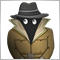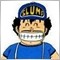# Compile error mt4 expression not boolean ?58

Can anyone help me ?

when I compile in mt4 strategy tester it says expression not boolean ? it used to say something like 0 errors 0 faults etc ?

It's got me, it still compiles the expert advisor ok but Its not confirming that there is no errors in the programme.

Geoff7

Assuming you mean MetaEditor, not Strategy Tester, but you probably used a single equal sign in a place where you need two.  Use a single = when assigning values and == when evaluating something in an expression. For example:

if(OrderType()==0  <--Compiler is happy

if(OrderType()=0 <-- Expression is not boolean error

If you post the line of code it's complaining about I'm sure we can find it quickly.587

Piptide:

Assuming you mean MetaEditor, not Strategy Tester, but you probably used a single equal sign in a place where you need two.  Use a single = when assigning values and == when evaluating something in an expression. For example:

if(OrderType()==0  <--Compiler is happy

if(OrderType()=0 <-- Expression is not boolean error

If you post the line of code it's complaining about I'm sure we can find it quickly.

Have the same problem,

here is my code:

```//--- Has the candle actually formed
bool ValidCandleCheck(string symbol, ENUM_TIMEFRAMES timeframe)
{
double low = iLow(symbol,timeframe,0);
double high = iHigh(symbol,timeframe,0);
if( MathAbs( high - low ) == 0 || low == 0 || high == 0 )
{
return(False);
}
else
{
return(True);
}
}```9225

```//--- Has the candle actually formed
bool ValidCandleCheck(string symbol, ENUM_TIMEFRAMES timeframe)
{
double low = iLow(symbol,timeframe,0);
double high = iHigh(symbol,timeframe,0);
if( MathAbs( high - low ) == 0 || low == 0 || high == 0 )
{
return(False);
}
else
{
return(True);
}
return(True);
}```
or
```//--- Has the candle actually formed
bool ValidCandleCheck(string symbol, ENUM_TIMEFRAMES timeframe)
{
double low = iLow(symbol,timeframe,0);
double high = iHigh(symbol,timeframe,0);
if( MathAbs( high - low ) == 0 || low == 0 || high == 0 )
{
return(False);
}
return(True);
}```

or

```//--- Has the candle actually formed
bool ValidCandleCheck(string symbol, ENUM_TIMEFRAMES timeframe)
{
double low = iLow(symbol,timeframe,0);
double high = iHigh(symbol,timeframe,0);

return(( MathAbs( high - low ) == 0 || low == 0 || high == 0 ) ? False : True);
}```21815

```//--- Has the candle actually formed
bool ValidCandleCheck(string symbol, ENUM_TIMEFRAMES timeframe)
{
double low = iLow(symbol,timeframe,0);
double high = iHigh(symbol,timeframe,0);

return !( MathAbs( high - low ) == 0 || low == 0 || high == 0 );
}```
or
```//--- Has the candle actually formed
bool ValidCandleCheck(string symbol, ENUM_TIMEFRAMES timeframe)
{
double low = iLow(symbol,timeframe,0);
double high = iHigh(symbol,timeframe,0);

return MathAbs( high - low ) != 0 && low != 0 && high != 0;
}```508

whroeder1:
or

Hi, something like this happens to me, with the new version I get a warning of "not boolean expression" in this code. Can you tell me what the right code is?

f ((UseFridayFinalTradeTime && (Hour()>=FridayFinalHourGMT + ServerTimeZone) && DayOfWeek()==5)||DayOfWeek()==0)return (False); // Friday ControlModerator
23389

You used to be able to use True and False with the capitalized initial letter

now you must use

true and false - all lower case.